# Detect and Recognize Car License Plate from a video in real time

Recognizing Car License Plate is a very important task for a camera surveillance-based security system. We can extract the license plate from an image using some computer vision techniques and then we can use Optical Character Recognition to recognize the license number. Here I will guide you through the whole procedure of this task.

Requirements:

opencv-python 3.4.2
numpy 1.17.2
skimage 0.16.2
tensorflow 1.15.0
imutils 0.5.3

Example:

Input: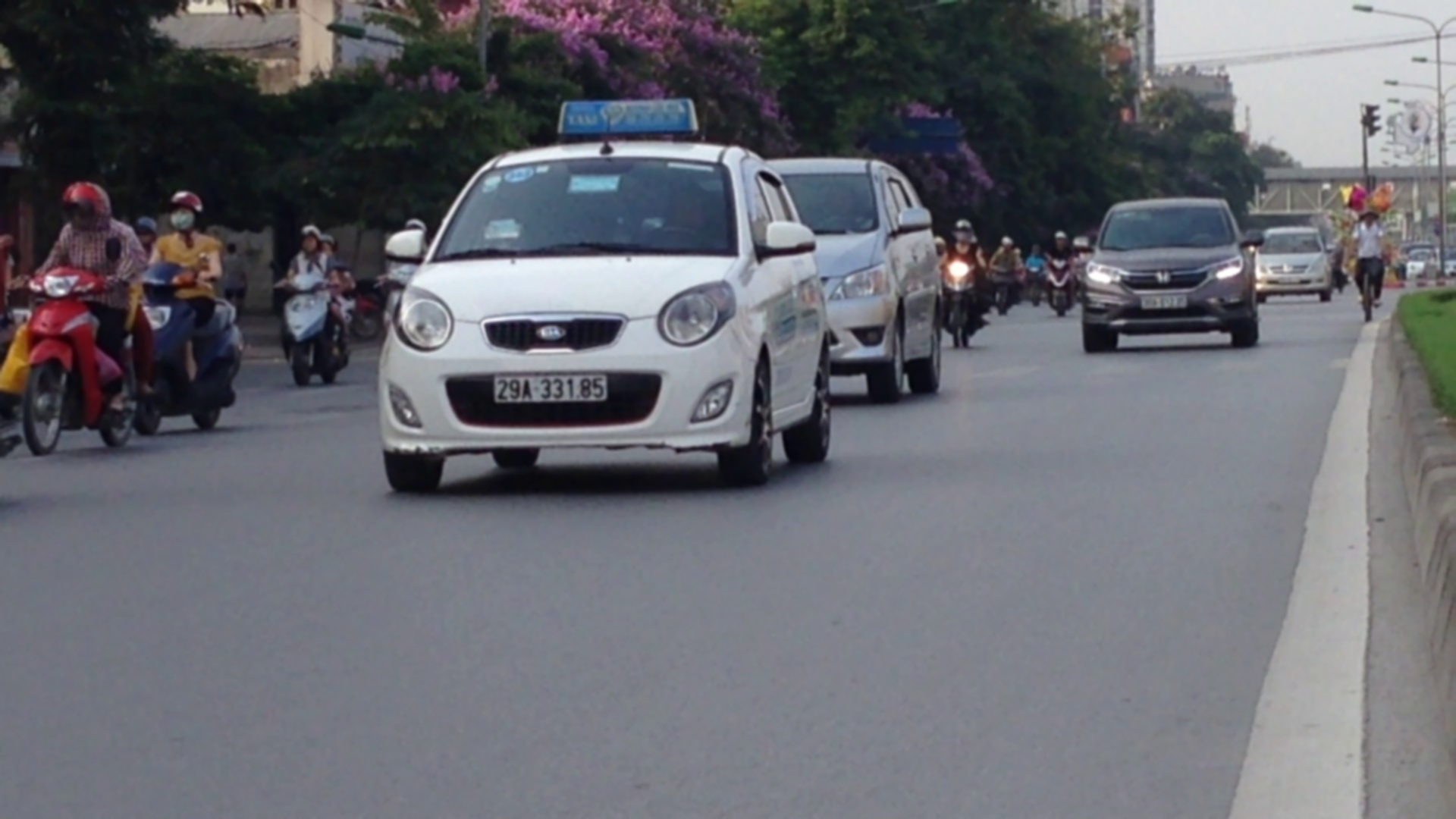Output:
29A33185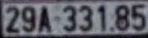Approach:

• Find all the contours in the image.
• Find the bounding rectangle of every contour.
• Compare and validate the sides ratio and area of every bounding rectangle with an average license plate.
• Apply image segmentation in the image inside validated contour to find characters in it.
• Recognize characters using an OCR.

Methodology:

1. To reduce the noise we need to blur the input Image with Gaussian Blur then convert the it to grayscale.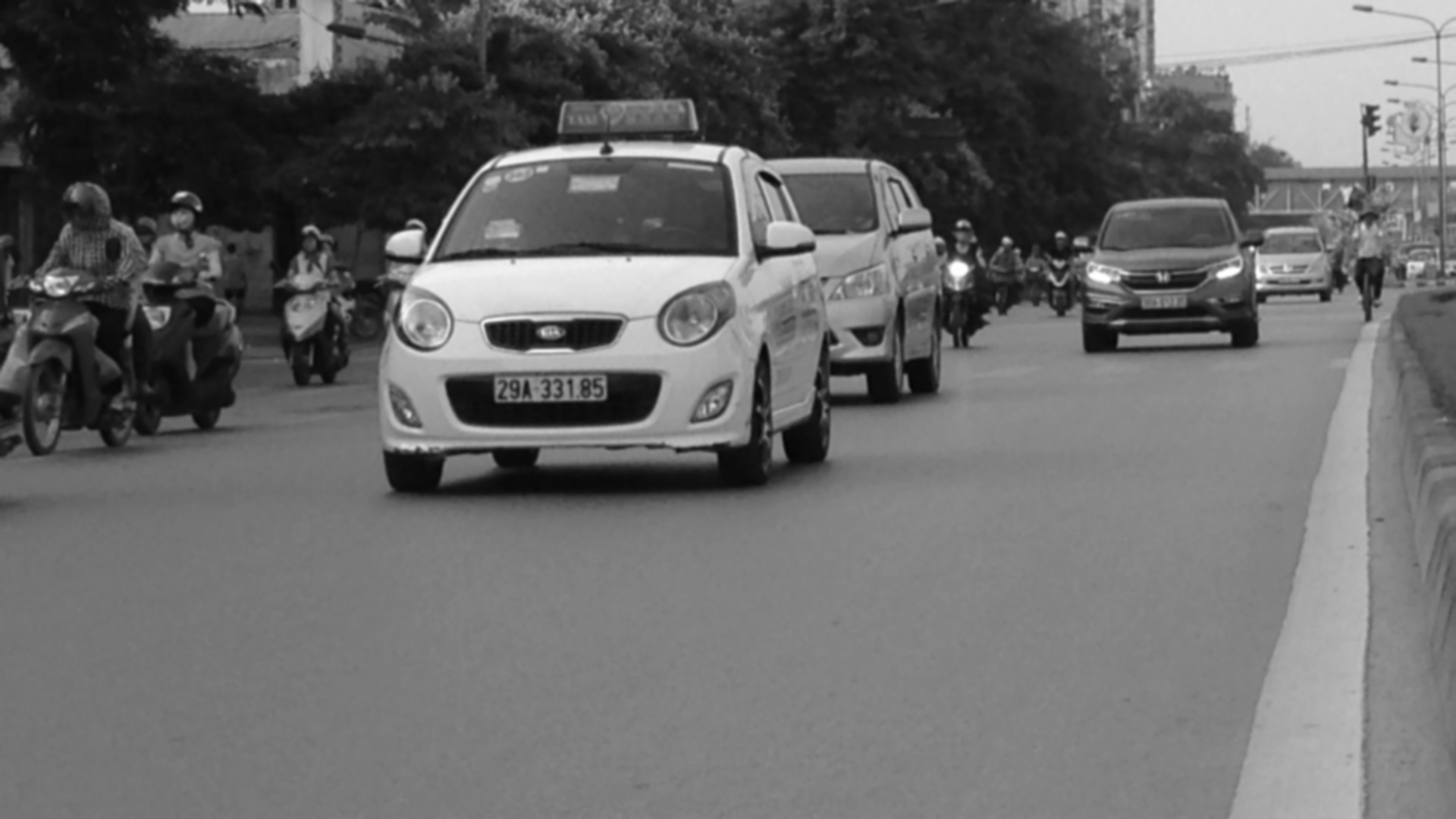2. Find vertical edges in the image.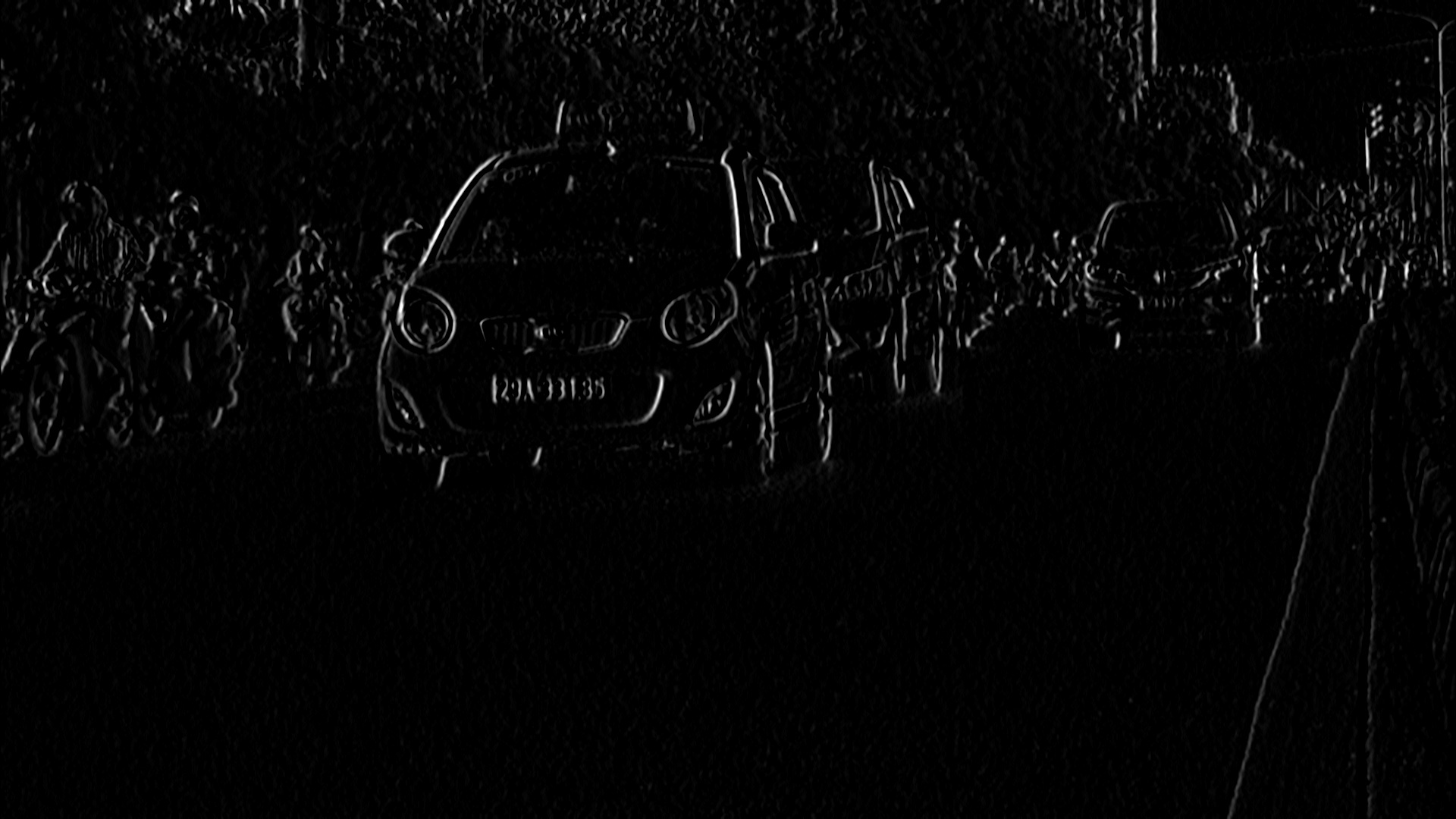3. To reveal the plate we have to binarize the image. For this apply Otsu’s Thresholding on the vertical edge image. In other thresholding methods we have to choose a threshold value to binarize the image but Otsu’s Thresholding determines the value automatically.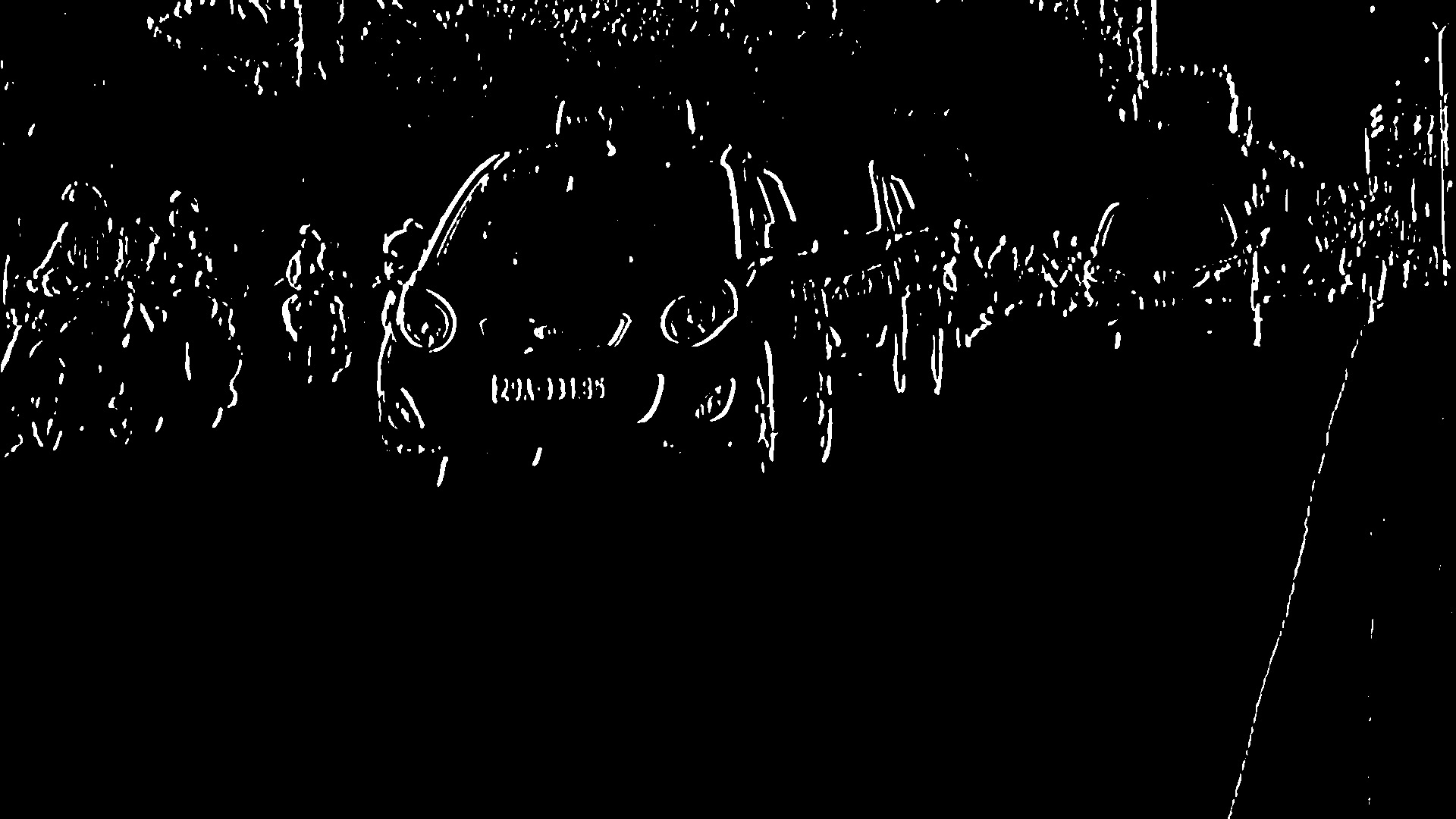4. Apply Closing Morphological Transformation on thresholded image. Closing is useful to fill small black regions between white regions in a thresholded image. It reveals the rectangular white box of license plate.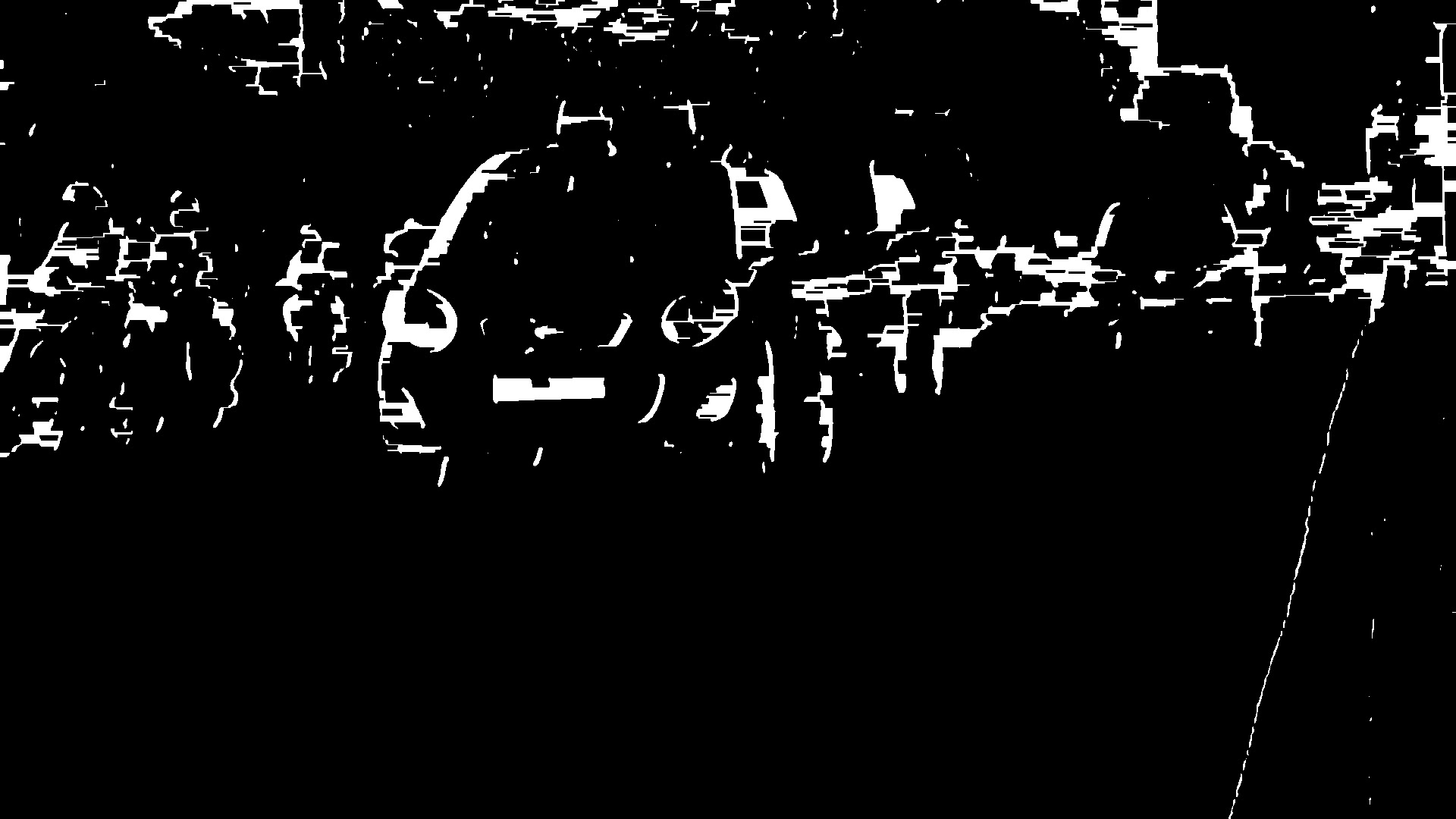5. Above 4 steps are performed by preprocess method of class PlateFinder

 `def` `preprocess(``self``, input_img): ` ` `  `    ``imgBlurred ``=` `cv2.GaussianBlur(input_img, (``7``, ``7``), ``0``) ` `     `  `    ``# convert to gray ` `    ``gray ``=` `cv2.cvtColor(imgBlurred, ` `                        ``cv2.COLOR_BGR2GRAY)  ` `     `  `    ``# sobelX to get the vertical edges ` `    ``sobelx ``=` `cv2.Sobel(gray, cv2.CV_8U,  ` `                       ``1``, ``0``, ksize ``=` `3``)   ` `     `  `     ``# otsu's thresholding ` `    ``ret2, threshold_img ``=` `cv2.threshold(sobelx,``0``, ``255``, ` `                           ``cv2.THRESH_BINARY ``+` `cv2.THRESH_OTSU) `   `    ``element ``=` `self``.element_structure ` `    ``morph_n_thresholded_img ``=` `threshold_img.copy() ` `    ``cv2.morphologyEx(src ``=` `threshold_img, ` `                     ``op ``=` `cv2.MORPH_CLOSE, ` `                     ``kernel ``=` `element,  ` `                     ``dst ``=` `morph_n_thresholded_img) ` `     `  `    ``return` `morph_n_thresholded_img `

6. To detect the plate we need to find contours in the image. It is important to binarize and morph the image before finding contours so that it can find more relevant and less number of contours in the image. If you draw all the extracted contours on original image, it would look like this: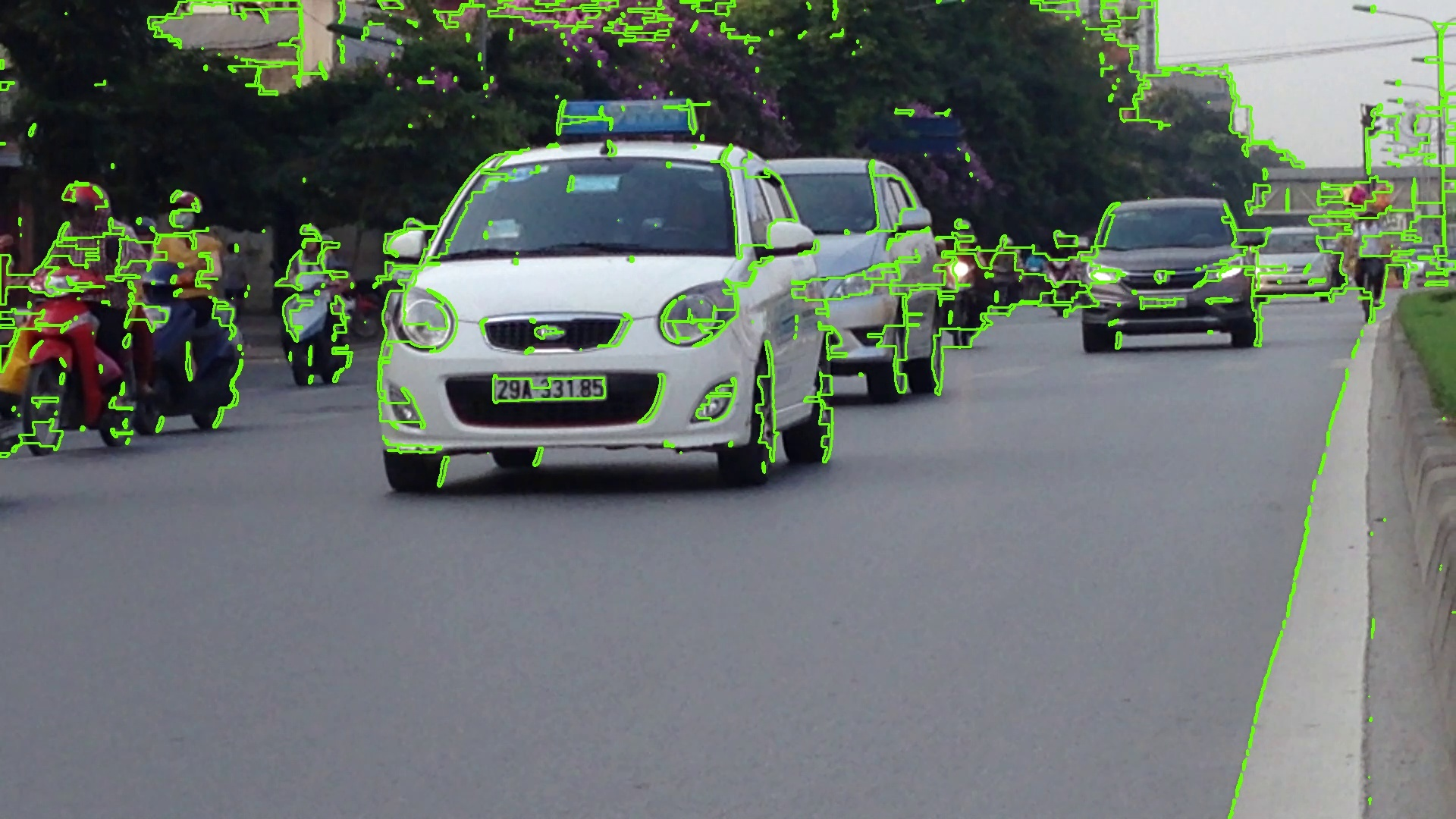7. This step is performed by extract_contours method of class PlateFinder

 `def` `extract_contours(``self``, after_preprocess): ` ` `  `    ``_, contours, _ ``=` `cv2.findContours(after_preprocess, ` `                                      ``mode ``=` `cv2.RETR_EXTERNAL, ` `                                      ``method ``=` `cv2.CHAIN_APPROX_NONE) ` `    ``return` `contours `

8. Now find the minimum area rectangle enclosed by each of the contour and validate their side ratios and area. We have defined the minimum and maximum area of the plate as 4500 and 30000 respectively.
9. Code: Methods validating the area and side ratios of minimum area rectangle are validateRatio and
preRatioCheck of class PlateFinder:

 `def` `validateRatio(``self``, rect): ` ` `  `    ``(x, y), (width, height), rect_angle ``=` `rect `   `    ``if` `(width > height): ` `        ``angle ``=` `-``rect_angle ` `         `  `    ``else``: ` `        ``angle ``=` `90` `+` `rect_angle `   `    ``if` `angle > ``15``: ` `        ``return` `False` `     `  `    ``if` `(height ``=``=` `0` `or` `width ``=``=` `0``): ` `        ``return` `False`   `    ``area ``=` `width ``*` `height ` `     `  `    ``if` `not` `self``.preRatioCheck(area, width, height): ` `        ``return` `False` `     `  `    ``else``: ` `        ``return` `True`   `def` `preRatioCheck(``self``, area, width, height): ` `     `  `    ``min` `=` `self``.min_area ` `    ``max` `=` `self``.max_area `   `    ``ratioMin ``=` `2.5` `    ``ratioMax ``=` `7`   `    ``ratio ``=` `float``(width) ``/` `float``(height) ` `     `  `    ``if` `ratio < ``1``: ` `        ``ratio ``=` `1` `/` `ratio `   `    ``if` `(area < ``min` `or` `area > ``max``) ``or` `(ratio < ratioMin ``or` `ratio > ratioMax): ` `        ``return` `False` `     `  `    ``return` `True`

10. Now find the contours in the validated region and validate the side ratios and area of the bounding rectangle of the largest contour in that region. After validating you will get a perfect contour of a license plate. Now extract that contour from the original image. You will get the image of plate:11. Code: This step is performed by clean_plate and ratioCheck method of class PlateFinder.

 `def` `clean_plate(``self``, plate): ` ` `  `    ``gray ``=` `cv2.cvtColor(plate, cv2.COLOR_BGR2GRAY) ` `    ``thresh ``=` `cv2.adaptiveThreshold(gray, ``255``,  ` `                                   ``cv2.ADAPTIVE_THRESH_GAUSSIAN_C, ` `                                   ``cv2.THRESH_BINARY, ``11``, ``2``) ` `     `  `    ``_, contours, _ ``=` `cv2.findContours(thresh.copy(),  ` `                                      ``cv2.RETR_EXTERNAL, ` `                                      ``cv2.CHAIN_APPROX_NONE) `   `    ``if` `contours: ` `         `  `        ``areas ``=` `[cv2.contourArea(c) ``for` `c ``in` `contours] ` `         `  `        ``# index of the largest contour in the  ` `        ``# areas array ` `        ``max_index ``=` `np.argmax(areas)   `   `        ``max_cnt ``=` `contours[max_index] ` `        ``max_cntArea ``=` `areas[max_index] ` `        ``x, y, w, h ``=` `cv2.boundingRect(max_cnt) `   `        ``if` `not` `self``.ratioCheck(max_cntArea, ` `                               ``plate.shape[``1``], ` `                               ``plate.shape[``0``]): ` `            ``return` `plate, ``False``, ``None` `         `  `        ``return` `plate, ``True``, [x, y, w, h] ` `     `  `    ``else``: ` `        ``return` `plate, ``False``, ``None`   `def` `ratioCheck(``self``, area, width, height): ` `     `  `    ``min` `=` `self``.min_area ` `    ``max` `=` `self``.max_area `   `    ``ratioMin ``=` `3` `    ``ratioMax ``=` `6`   `    ``ratio ``=` `float``(width) ``/` `float``(height) ` `     `  `    ``if` `ratio < ``1``: ` `        ``ratio ``=` `1` `/` `ratio `   `    ``if` `(area < ``min` `or` `area > ``max``) ``or` `(ratio < ratioMin ``or` `ratio > ratioMax): ` `        ``return` `False` `     `  `    ``return` `True`

12. To recognize the characters on license plate precisely, we have to apply image segmentation. For that first step is to extract the value channel from the HSV format of the plate’s image. It would look like-
13.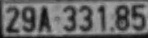14. Now apply adaptive thresholding on the plate’s value channel image to binarize it and reveal the characters. The image of plate can have different lightning conditions in different areas, in that case adaptive thresholding can be more suitable to binarize because it uses different threshold values for different regions based on the brightness of the pixels in the region around it.
15.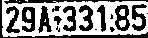16. After binarizing apply bitwise not operation on the image to find the connected components in the image so that we can extract character candidates.
17.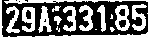18. Construct a mask to display all the character components and then find contours in mask. After extracting the contours take the largest one, find its bounding rectangle and validate side ratios.
19. After validating the side ratios find the convex hull of the contour ad draw it on the character candidate mask. The mask would look like-
20.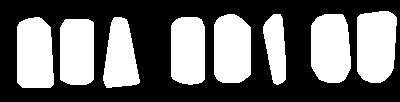21. Now find all the contours in the character candidate mask and extract those contour areas from the plate’s value thresholded image, you will get all the characters separately.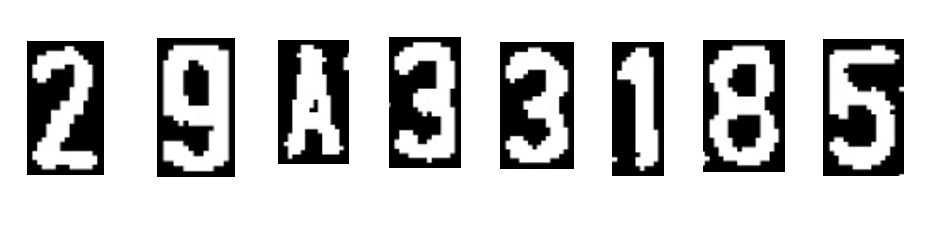Steps 8 to 13 are performed by segment_chars function that you can find below in the full source code. The driver code for the functions used in steps 6 to 13 is written in the method check_plate of class PlateFinder.
22. Now use OCR to recognize the character one by one.

Full Source Code with its working: First, create a PlateFinder class that finds the license plates and validates its size ratio and area.

 `import` `cv2 ` `import` `numpy as np ` `from` `skimage.filters ``import` `threshold_local ` `import` `tensorflow as tf ` `from` `skimage ``import` `measure ` `import` `imutils ` ` `  ` `  `def` `sort_cont(character_contours): ` `    ``""" ` `    ``To sort contours ` `    ``"""` `    ``i ``=` `0` `    ``boundingBoxes ``=` `[cv2.boundingRect(c) ``for` `c ``in` `character_contours] ` `     `  `    ``(character_contours, boundingBoxes) ``=` `zip``(``*``sorted``(``zip``(character_contours, ` `                                                          ``boundingBoxes), ` `                                                      ``key ``=` `lambda` `b: b[``1``][i], ` `                                                      ``reverse ``=` `False``)) ` `     `  `    ``return` `character_contours ` ` `  ` `  `def` `segment_chars(plate_img, fixed_width): ` `     `  `    ``""" ` `    ``extract Value channel from the HSV format ` `    ``of image and apply adaptive thresholding ` `    ``to reveal the characters on the license plate ` `    ``"""` `    ``V ``=` `cv2.split(cv2.cvtColor(plate_img, cv2.COLOR_BGR2HSV))[``2``] ` ` `  `    ``thresh ``=` `cv2.adaptiveThreshold(value, ``255``,  ` `                                   ``cv2.ADAPTIVE_THRESH_GAUSSIAN_C, ` `                                   ``cv2.THRESH_BINARY,  ` `                                   ``11``, ``2``) ` ` `  `    ``thresh ``=` `cv2.bitwise_not(thresh) ` ` `  `    ``# resize the license plate region to ` `    ``# a canoncial size ` `    ``plate_img ``=` `imutils.resize(plate_img, width ``=` `fixed_width) ` `    ``thresh ``=` `imutils.resize(thresh, width ``=` `fixed_width) ` `    ``bgr_thresh ``=` `cv2.cvtColor(thresh, cv2.COLOR_GRAY2BGR) ` ` `  `    ``# perform a connected components analysis  ` `    ``# and initialize the mask to store the locations ` `    ``# of the character candidates ` `    ``labels ``=` `measure.label(thresh, neighbors ``=` `8``, background ``=` `0``) ` ` `  `    ``charCandidates ``=` `np.zeros(thresh.shape, dtype ``=``'uint8'``) ` ` `  `    ``# loop over the unique components ` `    ``characters ``=` `[] ` `    ``for` `label ``in` `np.unique(labels): ` `         `  `        ``# if this is the background label, ignore it ` `        ``if` `label ``=``=` `0``: ` `            ``continue` `        ``# otherwise, construct the label mask to display ` `        ``# only connected components for the current label, ` `        ``# then find contours in the label mask ` `        ``labelMask ``=` `np.zeros(thresh.shape, dtype ``=``'uint8'``) ` `        ``labelMask[labels ``=``=` `label] ``=` `255` ` `  `        ``cnts ``=` `cv2.findContours(labelMask,  ` `                     ``cv2.RETR_EXTERNAL,  ` `                     ``cv2.CHAIN_APPROX_SIMPLE) ` ` `  `        ``cnts ``=` `cnts[``0``] ``if` `imutils.is_cv2() ``else` `cnts[``1``] ` ` `  `        ``# ensure at least one contour was found in the mask ` `        ``if` `len``(cnts) > ``0``: ` ` `  `            ``# grab the largest contour which corresponds  ` `            ``# to the component in the mask, then grab the ` `            ``# bounding box for the contour ` `            ``c ``=` `max``(cnts, key ``=` `cv2.contourArea) ` `            ``(boxX, boxY, boxW, boxH) ``=` `cv2.boundingRect(c) ` ` `  `            ``# compute the aspect ratio, solodity, and  ` `            ``# height ration for the component ` `            ``aspectRatio ``=` `boxW ``/` `float``(boxH) ` `            ``solidity ``=` `cv2.contourArea(c) ``/` `float``(boxW ``*` `boxH) ` `            ``heightRatio ``=` `boxH ``/` `float``(plate_img.shape[``0``]) ` ` `  `            ``# determine if the aspect ratio, solidity,  ` `            ``# and height of the contour pass the rules ` `            ``# tests ` `            ``keepAspectRatio ``=` `aspectRatio < ``1.0` `            ``keepSolidity ``=` `solidity > ``0.15` `            ``keepHeight ``=` `heightRatio > ``0.5` `and` `heightRatio < ``0.95` ` `  `            ``# check to see if the component passes ` `            ``# all the tests ` `            ``if` `keepAspectRatio ``and` `keepSolidity ``and` `keepHeight ``and` `boxW > ``14``: ` `                 `  `                ``# compute the convex hull of the contour ` `                ``# and draw it on the character candidates ` `                ``# mask ` `                ``hull ``=` `cv2.convexHull(c) ` ` `  `                ``cv2.drawContours(charCandidates, [hull], ``-``1``, ``255``, ``-``1``) ` ` `  `    ``_, contours, hier ``=` `cv2.findContours(charCandidates, ` `                                         ``cv2.RETR_EXTERNAL, ` `                                         ``cv2.CHAIN_APPROX_SIMPLE) ` `     `  `    ``if` `contours: ` `        ``contours ``=` `sort_cont(contours) ` `         `  `        ``# value to be added to each dimension  ` `        ``# of the character ` `        ``addPixel ``=` `4`   `        ``for` `c ``in` `contours: ` `            ``(x, y, w, h) ``=` `cv2.boundingRect(c) ` `            ``if` `y > addPixel: ` `                ``y ``=` `y ``-` `addPixel ` `            ``else``: ` `                ``y ``=` `0` `            ``if` `x > addPixel: ` `                ``x ``=` `x ``-` `addPixel ` `            ``else``: ` `                ``x ``=` `0` `            ``temp ``=` `bgr_thresh[y:y ``+` `h ``+` `(addPixel ``*` `2``), ` `                              ``x:x ``+` `w ``+` `(addPixel ``*` `2``)] ` ` `  `            ``characters.append(temp) ` `             `  `        ``return` `characters ` `     `  `    ``else``: ` `        ``return` `None` ` `  ` `  ` `  `class` `PlateFinder: ` `    ``def` `__init__(``self``): ` `         `  `        ``# minimum area of the plate ` `        ``self``.min_area ``=` `4500`   `         `  `        ``# maximum area of the plate ` `        ``self``.max_area ``=` `30000`   ` `  `        ``self``.element_structure ``=` `cv2.getStructuringElement( ` `                              ``shape ``=` `cv2.MORPH_RECT, ksize ``=``(``22``, ``3``)) ` ` `  `    ``def` `preprocess(``self``, input_img): ` `         `  `        ``imgBlurred ``=` `cv2.GaussianBlur(input_img, (``7``, ``7``), ``0``) ` `         `  `        ``# convert to gray ` `        ``gray ``=` `cv2.cvtColor(imgBlurred, cv2.COLOR_BGR2GRAY)  ` `         `  `        ``# sobelX to get the vertical edges ` `        ``sobelx ``=` `cv2.Sobel(gray, cv2.CV_8U, ``1``, ``0``, ksize ``=` `3``)   ` `         `  `        ``# otsu's thresholding ` `        ``ret2, threshold_img ``=` `cv2.threshold(sobelx, ``0``, ``255``, ` `                         ``cv2.THRESH_BINARY ``+` `cv2.THRESH_OTSU)  ` ` `  `        ``element ``=` `self``.element_structure ` `        ``morph_n_thresholded_img ``=` `threshold_img.copy() ` `        ``cv2.morphologyEx(src ``=` `threshold_img,  ` `                         ``op ``=` `cv2.MORPH_CLOSE, ` `                         ``kernel ``=` `element, ` `                         ``dst ``=` `morph_n_thresholded_img) ` `         `  `        ``return` `morph_n_thresholded_img ` ` `  `    ``def` `extract_contours(``self``, after_preprocess): ` `         `  `        ``_, contours, _ ``=` `cv2.findContours(after_preprocess,  ` `                                          ``mode ``=` `cv2.RETR_EXTERNAL, ` `                                          ``method ``=` `cv2.CHAIN_APPROX_NONE) ` `        ``return` `contours ` ` `  `    ``def` `clean_plate(``self``, plate): ` `         `  `        ``gray ``=` `cv2.cvtColor(plate, cv2.COLOR_BGR2GRAY) ` `        ``thresh ``=` `cv2.adaptiveThreshold(gray, ` `                                       ``255``,  ` `                                       ``cv2.ADAPTIVE_THRESH_GAUSSIAN_C,  ` `                                       ``cv2.THRESH_BINARY, ` `                                       ``11``, ``2``) ` `         `  `        ``_, contours, _ ``=` `cv2.findContours(thresh.copy(),  ` `                                          ``cv2.RETR_EXTERNAL, ` `                                          ``cv2.CHAIN_APPROX_NONE) ` ` `  `        ``if` `contours: ` `            ``areas ``=` `[cv2.contourArea(c) ``for` `c ``in` `contours] ` `             `  `            ``# index of the largest contour in the area ` `            ``# array ` `            ``max_index ``=` `np.argmax(areas)   ` ` `  `            ``max_cnt ``=` `contours[max_index] ` `            ``max_cntArea ``=` `areas[max_index] ` `            ``x, y, w, h ``=` `cv2.boundingRect(max_cnt) ` `            ``rect ``=` `cv2.minAreaRect(max_cnt) ` `             `  `            ``if` `not` `self``.ratioCheck(max_cntArea, plate.shape[``1``],  ` `                                                ``plate.shape[``0``]): ` `                ``return` `plate, ``False``, ``None` `             `  `            ``return` `plate, ``True``, [x, y, w, h] ` `         `  `        ``else``: ` `            ``return` `plate, ``False``, ``None` ` `  ` `  ` `  `    ``def` `check_plate(``self``, input_img, contour): ` `         `  `        ``min_rect ``=` `cv2.minAreaRect(contour) ` `         `  `        ``if` `self``.validateRatio(min_rect): ` `            ``x, y, w, h ``=` `cv2.boundingRect(contour) ` `            ``after_validation_img ``=` `input_img[y:y ``+` `h, x:x ``+` `w] ` `            ``after_clean_plate_img, plateFound, coordinates ``=` `self``.clean_plate( ` `                                                        ``after_validation_img) ` `             `  `            ``if` `plateFound: ` `                ``characters_on_plate ``=` `self``.find_characters_on_plate( ` `                                              ``after_clean_plate_img) ` `                 `  `                ``if` `(characters_on_plate ``is` `not` `None` `and` `len``(characters_on_plate) ``=``=` `8``): ` `                    ``x1, y1, w1, h1 ``=` `coordinates ` `                    ``coordinates ``=` `x1 ``+` `x, y1 ``+` `y ` `                    ``after_check_plate_img ``=` `after_clean_plate_img ` `                     `  `                    ``return` `after_check_plate_img, characters_on_plate, coordinates ` `         `  `        ``return` `None``, ``None``, ``None` ` `  ` `  ` `  `    ``def` `find_possible_plates(``self``, input_img): ` `         `  `        ``""" ` `        ``Finding all possible contours that can be plates ` `        ``"""` `        ``plates ``=` `[] ` `        ``self``.char_on_plate ``=` `[] ` `        ``self``.corresponding_area ``=` `[] ` ` `  `        ``self``.after_preprocess ``=` `self``.preprocess(input_img) ` `        ``possible_plate_contours ``=` `self``.extract_contours(``self``.after_preprocess) ` ` `  `        ``for` `cnts ``in` `possible_plate_contours: ` `            ``plate, characters_on_plate, coordinates ``=` `self``.check_plate(input_img, cnts) ` `             `  `            ``if` `plate ``is` `not` `None``: ` `                ``plates.append(plate) ` `                ``self``.char_on_plate.append(characters_on_plate) ` `                ``self``.corresponding_area.append(coordinates) ` ` `  `        ``if` `(``len``(plates) > ``0``): ` `            ``return` `plates ` `         `  `        ``else``: ` `            ``return` `None` ` `  `    ``def` `find_characters_on_plate(``self``, plate): ` ` `  `        ``charactersFound ``=` `segment_chars(plate, ``400``) ` `        ``if` `charactersFound: ` `            ``return` `charactersFound ` ` `  `    ``# PLATE FEATURES ` `    ``def` `ratioCheck(``self``, area, width, height): ` `         `  `        ``min` `=` `self``.min_area ` `        ``max` `=` `self``.max_area ` ` `  `        ``ratioMin ``=` `3` `        ``ratioMax ``=` `6` ` `  `        ``ratio ``=` `float``(width) ``/` `float``(height) ` `         `  `        ``if` `ratio < ``1``: ` `            ``ratio ``=` `1` `/` `ratio ` `         `  `        ``if` `(area < ``min` `or` `area > ``max``) ``or` `(ratio < ratioMin ``or` `ratio > ratioMax): ` `            ``return` `False` `         `  `        ``return` `True` ` `  `    ``def` `preRatioCheck(``self``, area, width, height): ` `         `  `        ``min` `=` `self``.min_area ` `        ``max` `=` `self``.max_area ` ` `  `        ``ratioMin ``=` `2.5` `        ``ratioMax ``=` `7` ` `  `        ``ratio ``=` `float``(width) ``/` `float``(height) ` `         `  `        ``if` `ratio < ``1``: ` `            ``ratio ``=` `1` `/` `ratio ` ` `  `        ``if` `(area < ``min` `or` `area > ``max``) ``or` `(ratio < ratioMin ``or` `ratio > ratioMax): ` `            ``return` `False` `         `  `        ``return` `True` ` `  `    ``def` `validateRatio(``self``, rect): ` `        ``(x, y), (width, height), rect_angle ``=` `rect ` ` `  `        ``if` `(width > height): ` `            ``angle ``=` `-``rect_angle ` `        ``else``: ` `            ``angle ``=` `90` `+` `rect_angle ` ` `  `        ``if` `angle > ``15``: ` `            ``return` `False` `         `  `        ``if` `(height ``=``=` `0` `or` `width ``=``=` `0``): ` `            ``return` `False` ` `  `        ``area ``=` `width ``*` `height ` `         `  `        ``if` `not` `self``.preRatioCheck(area, width, height): ` `            ``return` `False` `        ``else``: ` `            ``return` `True`

Here is the explanation of each and every method of PlateFinder class.
In preprocess method, following step has been done:

• Blur the Image
• Convert to Grayscale
• Find vertical edges
• Threshold the vertical edged image.
• Close Morph the Threshold image.

Method extract_contours returns all external contours from the preprocessed image.
Method find_possible_plates precprocess the image with preprocess method then extracts contours by extract_contours method then it checks side ratios and area of all extracted contours and cleans the image inside the contour with check_plate and clean_plate methods. After cleaning the contour image with clean_plate method, it finds all characters on the plate with find_characters_on_plate method.
find_characters_on_plate method uses segment_chars function to find the characters. It finds characters by computing the convex hull of the contours of a thresholded value image and drawing it on the characters to reveal them.
Code: Make another class to initialize Neural Network to predict the characters on the extracted license plate.

 `class` `OCR: ` `     `  `    ``def` `__init__(``self``): ` `         `  `        ``self``.model_file ``=` `"./model / binary_128_0.50_ver3.pb"` `        ``self``.label_file ``=` `"./model / binary_128_0.50_labels_ver2.txt"` `        ``self``.label ``=` `self``.load_label(``self``.label_file) ` `        ``self``.graph ``=` `self``.load_graph(``self``.model_file) ` `        ``self``.sess ``=` `tf.Session(graph ``=` `self``.graph) ` ` `  `    ``def` `load_graph(``self``, modelFile): ` `         `  `        ``graph ``=` `tf.Graph() ` `        ``graph_def ``=` `tf.GraphDef() ` `         `  `        ``with ``open``(modelFile, ``"rb"``) as f: ` `            ``graph_def.ParseFromString(f.read()) ` `         `  `        ``with graph.as_default(): ` `            ``tf.import_graph_def(graph_def) ` `         `  `        ``return` `graph ` ` `  `    ``def` `load_label(``self``, labelFile): ` `        ``label ``=` `[] ` `        ``proto_as_ascii_lines ``=` `tf.gfile.GFile(labelFile).readlines() ` `         `  `        ``for` `l ``in` `proto_as_ascii_lines: ` `            ``label.append(l.rstrip()) ` `         `  `        ``return` `label ` ` `  `    ``def` `convert_tensor(``self``, image, imageSizeOuput): ` `        ``""" ` `        ``takes an image and tranform it in tensor ` `        ``"""` `        ``image ``=` `cv2.resize(image,  ` `                           ``dsize ``=``(imageSizeOuput, ` `                                  ``imageSizeOuput), ` `                           ``interpolation ``=` `cv2.INTER_CUBIC) ` `         `  `        ``np_image_data ``=` `np.asarray(image) ` `        ``np_image_data ``=` `cv2.normalize(np_image_data.astype(``'float'``), ` `                                      ``None``, ``-``0.5``, .``5``, ` `                                      ``cv2.NORM_MINMAX) ` `         `  `        ``np_final ``=` `np.expand_dims(np_image_data, axis ``=` `0``) ` `         `  `        ``return` `np_final ` ` `  `    ``def` `label_image(``self``, tensor): ` ` `  `        ``input_name ``=` `"import / input"` `        ``output_name ``=` `"import / final_result"` ` `  `        ``input_operation ``=` `self``.graph.get_operation_by_name(input_name) ` `        ``output_operation ``=` `self``.graph.get_operation_by_name(output_name) ` ` `  `        ``results ``=` `self``.sess.run(output_operation.outputs[``0``], ` `                                ``{input_operation.outputs[``0``]: tensor}) ` `        ``results ``=` `np.squeeze(results) ` `        ``labels ``=` `self``.label ` `        ``top ``=` `results.argsort()[``-``1``:][::``-``1``] ` `         `  `        ``return` `labels[top[``0``]] ` ` `  `    ``def` `label_image_list(``self``, listImages, imageSizeOuput): ` `        ``plate ``=` `"" ` `         `  `        ``for` `img ``in` `listImages: ` `             `  `            ``if` `cv2.waitKey(``25``) & ``0xFF` `=``=` `ord``(``'q'``): ` `                ``break` `            ``plate ``=` `plate ``+` `self``.label_image(``self``.convert_tensor(img, imageSizeOuput)) ` `         `  `        ``return` `plate, ``len``(plate) `

It loads the pretrained OCR model and its label file in load_graph and load_label functions. label_image_list method transforms the image to tensor with convert_tensor method and then predicts the label of tensor with label_image_list function and returns the license number.
Code: Create a main function to perform the whole task in a sequence.

 `if` `__name__ ``=``=` `"__main__"``: ` `     `  `    ``findPlate ``=` `PlateFinder() ` `    ``model ``=` `OCR() ` ` `  `    ``cap ``=` `cv2.VideoCapture(``'test_videos / video.MOV'``) ` `     `  `    ``while` `(cap.isOpened()): ` `        ``ret, img ``=` `cap.read() ` `         `  `        ``if` `ret ``=``=` `True``: ` `            ``cv2.imshow(``'original video'``, img) ` `             `  `            ``if` `cv2.waitKey(``25``) & ``0xFF` `=``=` `ord``(``'q'``): ` `                ``break` `             `  `            ``possible_plates ``=` `findPlate.find_possible_plates(img) ` `             `  `            ``if` `possible_plates ``is` `not` `None``: ` `                 `  `                ``for` `i, p ``in` `enumerate``(possible_plates): ` `                    ``chars_on_plate ``=` `findPlate.char_on_plate[i] ` `                    ``recognized_plate, _ ``=` `model.label_image_list( ` `                               ``chars_on_plate, imageSizeOuput ``=` `128``) ` ` `  `                    ``print``(recognized_plate) ` `                    ``cv2.imshow(``'plate'``, p) ` `                     `  `                    ``if` `cv2.waitKey(``25``) & ``0xFF` `=``=` `ord``(``'q'``): ` `                        ``break` `        ``else``: ` `            ``break` `             `  `    ``cap.release() ` `    ``cv2.destroyAllWindows() `

You can download the source code with OCR model and testing video from my GitHub .
How to improve the model?

• You can set a particular small region in the frame to find the plates inside it.(make sure all vehicle must pass through that region).
• You can train your own machine learning model to recognize characters because the given model doesn’t recognize all the alphabets.

References:
Automatic Number Plate Recognition System (ANPR): A Survey by Chirag Indravadanbhai Patel.
Image preprocessing techniques in OpenCV documentation.

Attention geek! Strengthen your foundations with the Python Programming Foundation Course and learn the basics.

To begin with, your interview preparations Enhance your Data Structures concepts with the Python DS Course.

My Personal Notes arrow_drop_up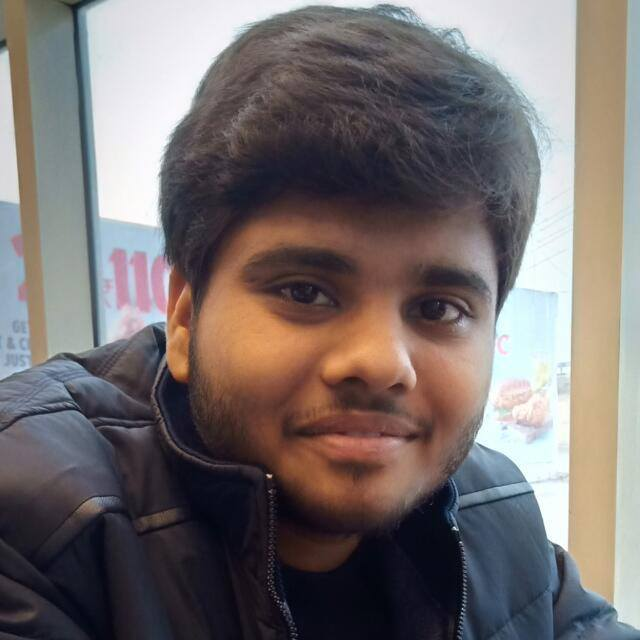Check out this Author's contributed articles.

If you like GeeksforGeeks and would like to contribute, you can also write an article using contribute.geeksforgeeks.org or mail your article to contribute@geeksforgeeks.org. See your article appearing on the GeeksforGeeks main page and help other Geeks.

Please Improve this article if you find anything incorrect by clicking on the "Improve Article" button below.

Article Tags :

1

Please write to us at contribute@geeksforgeeks.org to report any issue with the above content.